# Standard Deviation Formula## Standard Deviation Formula

To understand the standard deviation formula let us first understand the meaning of standard deviation. For a set of data the measure of dispersion about mean when expressed as the positive square root of the variance is called standard deviation. It is denoted by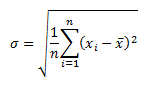For discrete frequency distribution of the type: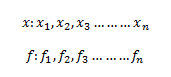The standard deviation is given as: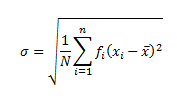Where $\overline{x}$  is the mean of the distribution and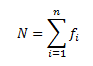For continuous frequency distribution, the mid-point of each class is considered for calculating the standard deviation. If frequency distribution of n classes is defined by its mid-point xi with frequency fi, the standard deviation is given by: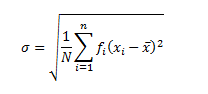Where $\overline{x}$  is the mean of the distribution and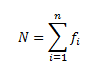Now let us try to obtain another standard deviation formula: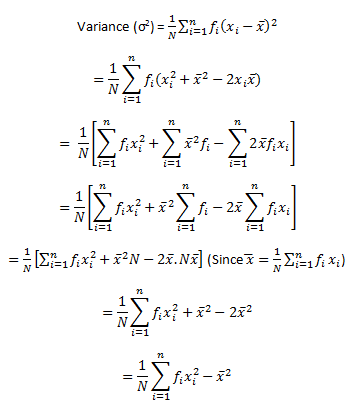Or,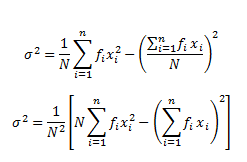This gives us the standard deviation (σ) as: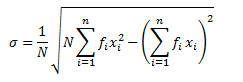This is the standard deviation formula for a given set of observations. But sometimes it happens that the value xi in a given data set or the midpoints of classes in a given frequency distribution is very enormous. In such cases, determination of mean or median or variance becomes hefty and time-consuming. To solve this problem we make use of step deviation method for simplifying the procedure.

Let us discuss this shortcut method for determining variance and standard deviation.

Let us consider the assumed mean as ‘A’ and the width of the class interval be h. Let the step deviation be yi.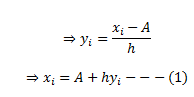The mean of a data set is given by: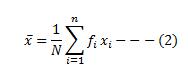Substituting the values of xi from equation (1) into equation (2), we get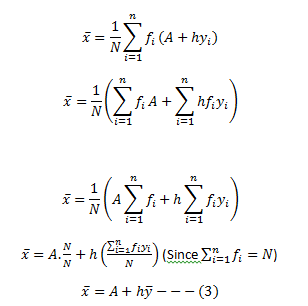Variance of the variable x,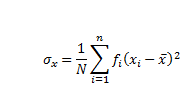Substituting the values from equation (1) and (3), we have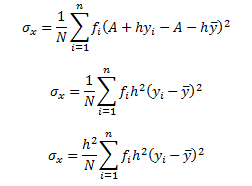σx=h2 × variance of variable yi

⇒σx2=h2σy2

⇒σx=hσy—(4)

From equation (3) and (4), we can conclude that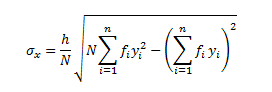Let us look into an example for a better insight.

Example: Find out the mean, variance and standard deviation for the following data representing the age group of employees working in XYZ Company.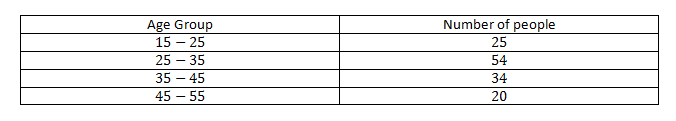Solution: Let the assumed mean A = 30 and h = 10. From the table given above, we can obtain.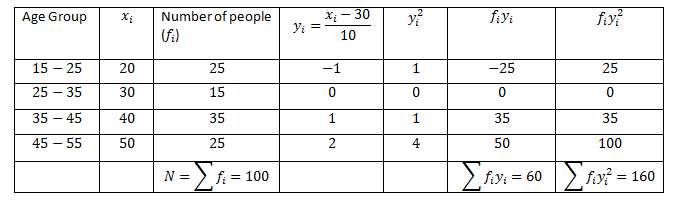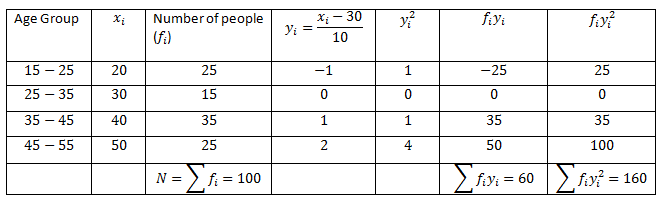Thus,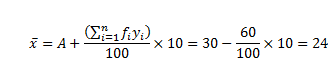The variance of the above data can be calculated as: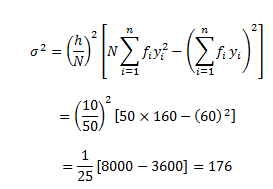The standard deviation can be given as:

σ=√176=13.266